Engineering Mechanics - Internal Forces

Why Engineering Mechanics Internal Forces?

In this section you can learn and practice Engineering Mechanics Questions based on "Internal Forces" and improve your skills in order to face the interview, competitive examination and various entrance test (CAT, GATE, GRE, MAT, Bank Exam, Railway Exam etc.) with full confidence.

Where can I get Engineering Mechanics Internal Forces questions and answers with explanation?

IndiaBIX provides you lots of fully solved Engineering Mechanics (Internal Forces) questions and answers with Explanation. Solved examples with detailed answer description, explanation are given and it would be easy to understand. All students, freshers can download Engineering Mechanics Internal Forces quiz questions with answers as PDF files and eBooks.

Where can I get Engineering Mechanics Internal Forces Interview Questions and Answers (objective type, multiple choice)?

Here you can find objective type Engineering Mechanics Internal Forces questions and answers for interview and entrance examination. Multiple choice and true or false type questions are also provided.

How to solve Engineering Mechanics Internal Forces problems?

You can easily solve all kind of Engineering Mechanics questions based on Internal Forces by practicing the objective type exercises given below, also get shortcut methods to solve Engineering Mechanics Internal Forces problems.

Exercise :: Internal Forces - General Questions

• Internal Forces - General Questions
1.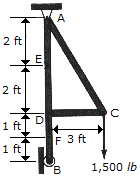Determine the internal axial force, shear force, and moment at point F of the frame.

 A. AF = 0, VF = 750 lb, MF = 750 lb-ft B. AF = 1500 lb, VF = 0, MF = 4500 lb-ft C. AF = 0, VF = 1500 lb, MF = 4500 lb-ft D. AF = 750 lb, VF = 0, MF = 750 lb-ft

Explanation:

No answer description available for this question. Let us discuss.

2.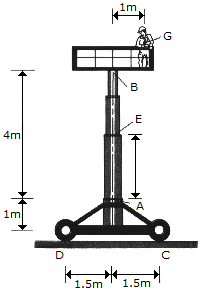The work platform supports an 80-kg man having a mass center at G. Determine the axial force, shear force, and bending moment at point E of the telescopic column AB due to the load of the man only.

 A. AE = 785 N C, VE = 0, ME = 785 N-m B. AE = 785 N C, VE = 392 N, ME = 0 C. AE = 785 N T, VE = 392 N, ME = 0 D. AE = 785 N T, VE = 0, ME = 785 N-m

Explanation:

No answer description available for this question. Let us discuss.

3.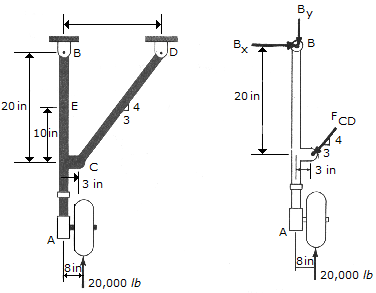Determine the internal axial force, shear force, and moment at point E of the oleo strut AB of the aircraft landing gear.

 A. AE = 9.33 kip C, VE = 8.00 kip, ME = 80.0 kip-in. B. AE = 11.11 kip T, VE = 6.67 kip, ME = 66.7 kip-in. C. AE = 9.33 kip T, VE = 8.00 kip, ME = 80.0 kip-in. D. AE = 11.11 kip C, VE = 6.67 kip, ME = 66.7 kip-in.

Explanation:

No answer description available for this question. Let us discuss.

4.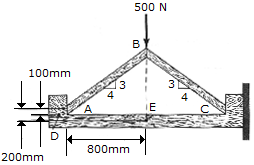A force of 500 N acts at the top of the two-member frame. If the members are in smooth contact with one another at A, B, and C with no fasteners, determine the shear force developed at a horizontal section through point D of the support. Also, what are the axial force, shear force and moment at point E?

 A. VD = 250 N, AE = 250 N, VE = 333 N, ME = 316 N-m B. VD = 333 N, AE = 333 N, VE = 250 N, ME = 267 N-m C. VD = 333 N, AE = 333 N, VE = 250 N, ME = 200 N-m D. VD = 250 N, AE = 250 N, VE = 333 N, ME = 267 N-m

Explanation:

No answer description available for this question. Let us discuss.

5.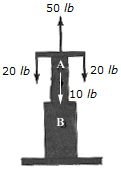The axial forces act on the shaft as shown. Determine the internal axial force at points A and B.

 A. AA = 0, AB = 10 lb C B. AA = 0, AB = 10 lb T C. AA = 10 lb C, AB = 0 D. AA = 10 lb T, AB = 0